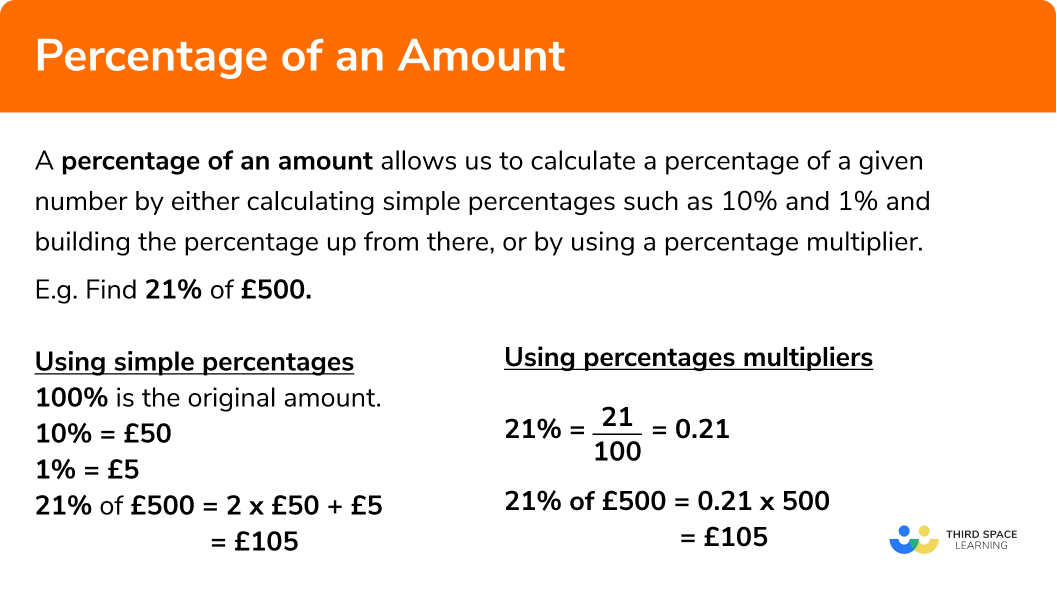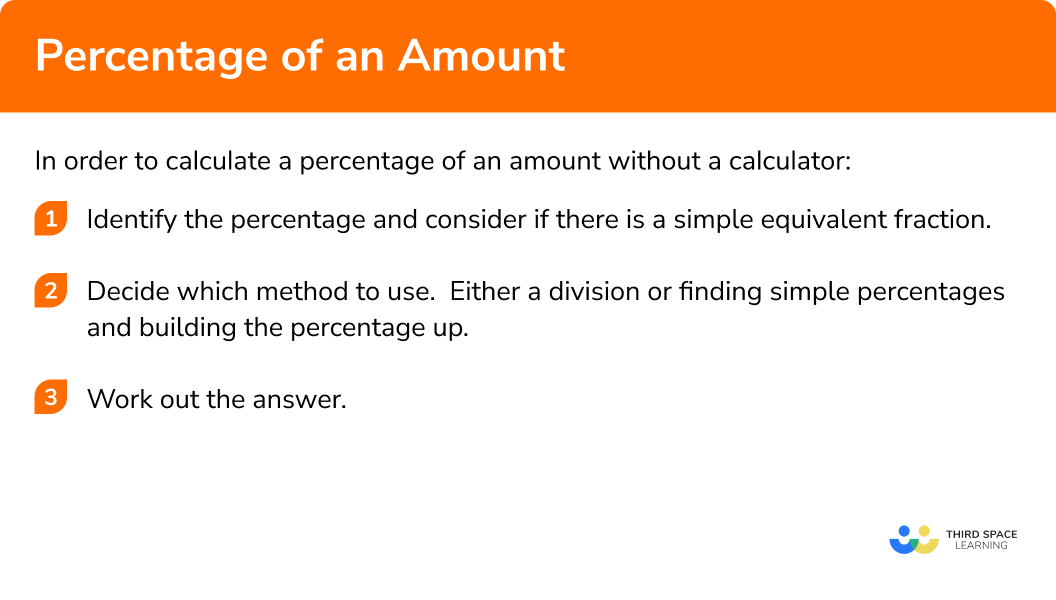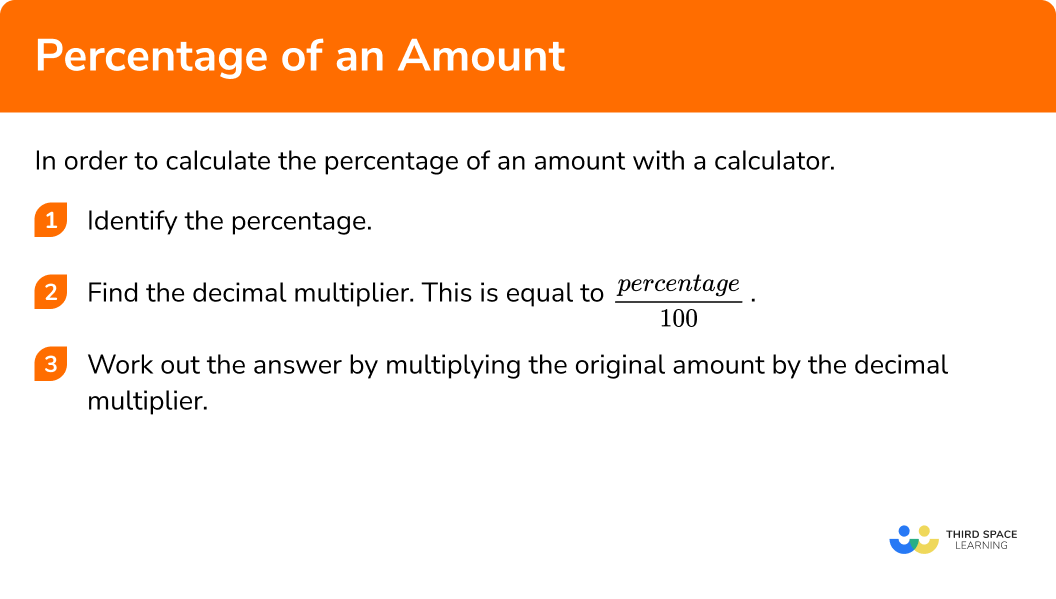GCSE Maths Number FDP Percentages

Percentage of an Amount

# Percentage Of An Amount

Here we will learn how to find percentage of an amount with and without using a calculator.

There are also percentage of an amount worksheets based on Edexcel, AQA and OCR exam questions, along with further guidance on where to go next if you’re still stuck.

## What is a percentage of an amount?

A percentage of an amount can be calculated by writing the percentage as a decimal or a fraction and then multiplying it by the amount. There are different methods you can use to calculate a percentage of a quantity.

For example, what is 35% of 60?

Method 1: The one percent method

Find 1% first by dividing the amount by 100 and then multiply this by the amount.

\frac{60}{100} \times 35 = 21

Method 2: The decimal multiplier method

Write the percentage as a decimal and then multiply the amount by this decimal.

35\%=0.35

60 \times 0.35=21

Method 3: Using equivalent fractions

Write the percentage as a fraction in its simplest form and then multiply the amount by this fraction.

35\% = \frac{35}{100} =\frac{7}{20}

60 \times \frac{7}{20} = \frac{60\times 7}{20} = \frac{420}{20} =\frac{42}{2}= 21

Alternatively you can calculate the fraction of the quantity like this,

35\% of 60 = \frac{7}{20} of 60

\frac{1}{20} of 60 = 60 \div 20 = 3

\frac{7}{20} of 60 = 60 \div 20 \times 7 = 21

Method 4: Building up an answer from simple percentages

Using simple percentages, you can build up to the answer to the question.

This question could be broken down into the following steps.

10\% of 60 = 6 (To find 10% we divide by 10)

5\% of 60 = 3 (5% is half of 10%)

30\% of 60 = 18 (30% is three lots of 10%)

35\% of 60 = 18+3=21 (30% plus 5%)

Methods 1 and 2 lend themselves to questions where a calculator is allowed. Methods 3 and 4 lend themselves to questions where calculators are not permitted.

### What is a percentage of an amount?## Amount as a percentage

You may be asked to express an amount as a percentage of another.

For example, express 40 as a percentage of 50.

This would mean writing the numbers as a fraction, and multiplying by 100. The first amount is the numerator and the second amount is the denominator.

\frac{40}{50}\times 100=80%

A different question could ask you to write 50 as a percentage of 20. Here it is very important to make sure that the first amount is the numerator and the second amount is the denominator.

\frac{50}{20}\times 100=250%

## How to find the percentage of an amount (non-calculator)

In order to find the percentage of an amount without a calculator:

1. Identify the percentage and consider if there is a simple equivalent fraction.
2. Decide which method to use.  Either a division or finding simple percentages and building it up.

### Explain how to calculate a percentage of an amount without a calculator in 3 steps## Percentage of an amount examples

### Example 1: percentage has a simple equivalent fraction

Find 25% of 120 km.

1. Identify the percentage.

$25\%=\frac{25}{100}=\frac{1}{4}$

2 Decide which method to use.  Either a division or finding 10%, 1% and building it up.

Since 25% = ¼, to find 25% we can divide by 4.

$120\div 4 = 30$

### Example 2: percentage has a simple equivalent fraction

Find 10% of 450 kg.

$10\%=\frac{10}{100}=\frac{1}{10}$

Since 10% = ⅟₁₀, to find 10% we can divide by 10.

$450\div 10 = 45$

### Example 3: percentage can be made up from 10% and 1%

Find 16% of £300.

$16\%= 10\% + 6\times 1\%$

Since 10% = ⅟₁₀, to find 10% we can divide by 10.

$300\div 10 = 30$

Then we divide by 10 again to find 1%.

$30\div 10 = 3$

$16\%= 10\% + 6\times 1\%$

Therefore,

16% of 300 is

$30 + (6\times 3) = 30 + 18 = 48$

### Example 4: percentage can be made up from 10% and 1%

Find 23% of £700.

$23\%= 2\times 10\% + 3\times 1\%$

Since 10% = ⅟₁₀, to find 10% we can divide by the denominator 10.

$700\div 10 = 70$

Then we divide by 10 again to find 1%.

$70\div 10 = 7$

$23\%= 2\times 10\% + 3\times 1\%$

Therefore,

23% of 700 is

$(2 × 70) + 3\times 7 = 140 + 21 = 161$

## How to find the percentage of an amount (with calculator)

In order to the percentage of an amount with a calculator:

1. Identify the percentage.
2. Find the decimal multiplier.
3. Work out the answer by multiplying the original amount by the decimal multiplier.

### Explain how to calculate the percentage of an amount with a calculator in 3 stepsStep by step guide: Percentage Multipliers

### Example 5: when you have a calculator

Work out 78% of £411.

$78\%$

Let’s find the decimal equivalent of 78%:

$78\%=\frac{78}{100}=0.78$

We can calculate this by dividing the numerator of 78 by the denominator of 100.

Writing 78% in its decimal form is 0.78.

$411 \times 0.78=320.58$

### Example 6: when you have a calculator

Work out 61% of £728.

$61\%$

$61\%=\frac{61}{100}=0.61$

We can calculate this by dividing the numerator of 61 by a hundred.

$728 \times 0.61=444.08$

### Common misconceptions

• Money and two decimal places

E.g.
An answer of £16.5 should be written as £16.50.  There are two digits needed for the pence.

• Using a non-calculator method when you have a calculator

If you are asked to find 29% of £391 you can do it by finding 10% and 1% and building it up to find 20% and 9% and adding them together. However it is much more simpler here to use a multiplier.

E.g.
Calculate 29% of £391

$391 \times 0.29=£ 113.39$

• Dividing by 10 – taking care with the decimal points

E.g.
Find 41% of 53 kg:

10%
is:

$53\div 10 = 5.3$

1% is:

$5.3\div 10 = 0.53$

41% of £53 is:

$(4 × 5.3) + 0.53 = 21.73$

Percentage of an amount is part of our series of lessons to support revision on percentages. You may find it helpful to start with the main percentages lesson for a summary of what to expect, or use the step by step guides below for further detail on individual topics. Other lessons in this series include:

### Practice percentage of an amount questions

1. Find 25\% of \pounds 280 without a calculator

\pounds 140\pounds 28\pounds 240\pounds 7025\% is the same as \frac{1}{4},

so we can calculate

\pounds 280 \div 4 = \pounds 70

2. Find 50\% of 70 kg without a calculator

35kg7kg17.5kg50kg50\% is the same as \frac{1}{2},

so we can calculate

70 \div 2 = 35

3. Find 12\% of 200g without a calculator

40g24g16.67g25g10\% of  200:

200 \div 10 = 20

1\% of  200:

200 \div 100 = 2

\begin{aligned} 12\%&=10\%+(2 \times 1\%)\\\\ &=20+(2 \times 2)\\\\ &=24 \end{aligned}

4. Find 27\% of 300 km without a calculator

75km81km67km27km10\% of  300km:

300 \div 10 = 30

1\% of  300km:

300 \div 100 = 3

\begin{aligned} 27\%&=(2 \times 10\%) + (7 \times 1\%)\\\\ &=(2 \times 30) + (7 \times 3)\\\\ &=60+21\\\\ &=81km \end{aligned}

5. Find 57\% of \pounds 710 with a calculator

\pounds 404.70\pounds 404.07\pounds 12.46\pounds 57057\%=\frac{57}{100} = 0.57

£710 \times 0.57 = \pounds 404.70

6. Find 83\% of \pounds 179 with a calculator

\pounds 30.43\pounds 83.79\pounds 148.57\pounds 143.2083\%=\frac{83}{100}=0.83

£179 \times 0.83 = \pounds 148.57

### Percentage of an amount GCSE questions

1. Without a calculator,

work out 20\% of 600 g.

(2 marks)

for finding 10\%,

600 ÷ 10 = 60

(1)

120 g

(1)

2. Andy is paid £1400 per month. He gets a 2 % increase in his monthly earnings. How much money will Andy earn per month after the increase?

(2 marks)

2\% = £28,

1400 + 28

(1)

£1428

(1)

3. At a shop T-shirts cost £4.60 each. If you buy 20 or more T-shirts, you can get 10\% off the total cost of all of the T-Shirts you have bought.

Work out the total cost of buying 26 T-shirts.

(3 Marks)

26 × £4.60 = £119.60

(1)

10\% of £119.60=£11.96 ,

(1)

£119.60 – £11.96 = 107.64

(1)

## Learning checklist

You have now learned how to:

• Interpret percentages as a fraction.
• Interpret percentages as a decimal.
• Interpret percentage multiplicatively.
• Use a calculator to calculate results accurately.

## Still stuck?

Prepare your KS4 students for maths GCSEs success with Third Space Learning. Weekly online one to one GCSE maths revision lessons delivered by expert maths tutors.

Find out more about our GCSE maths tuition programme.